# Impact of Second Order Slip and Non-uniform Suction on Non-linear Stagnation Point Flow of Alumina-water Nanofluid over Electromagnetic Sheet

Impact of Second Order Slip and Non-uniform Suction on Non-linear Stagnation Point Flow of Alumina-water Nanofluid over Electromagnetic Sheet

Manoj Kumar NayakAhmad Zeeshan | Zeshan Pervaiz Oluwole Daniel Makinde

Department of Physics, Radhakrishna Institute of Technology and Engineering, Bhubaneswar 752057, Odisha, India

Department of Mathematics and Statistics, International Islamic University, Islamabad, Pakistan

Faculty of Military Science, Stellenbosch University, Private Bag X2, Saldanha 7395, South Africa

Corresponding Author Email:
mkn2122@gmail.com
Page:
33-41
|
DOI:
https://doi.org/10.18280/mmc_b.880105
9 January 2019
|
Accepted:
20 March 2019
|
Published:
31 March 2019
| Citation

OPEN ACCESS

Abstract:

The purpose of the present article is to study the influence of second order slip and variable suction on non-linear stagnation point flow of Alumina-water nanofluid past an electromagnetic sheet embedded in a porous medium. A simulation model was established through hybrid Homotopy Analysis Method (HAM) and Genetic Algorithm Method (GAM). Through this it was found that favorable pressure gradient and modified Hartmann number yield accelerated fluid motion while porous matrix and first order slip result in decelerated flow over stationary/moving electromagnetic sheet. The finding of this research may serve as greater cooling agent due to more heat transfer rate from the electromagnetic sheet subject to favorable pressure gradient.

Keywords:

prescribed heat flux model, slip flow, permeable stretched sheet, HAM and GAM, porous medium

1. Introduction

In a spirit of broadening approach, the study of flow and heat transfer of nanofluids brings a lot of attention to numerous vibrant researchers because of its significant applications involving modern technology in diversified areas of need. The precious and inevitable applications of nanofluid flow and heat transfer include microchips cooling in computer processors, mirco-electromechanical systems (MEMS), obtaining fast transient aspect in heating systems, nuclear reactors, transportation, biomedicine, food and chemical industries, electronics/transformer/ vehicle cooling and developing the best quality lubricants and oils. Such credibility of nanofluids providing huge beneficiaries is only due to enhancement in its thermal conductivity. It is ironic that Choi  investigated experimentally and found that the addition of nanoparticles in the convectional heat transfer base fluids enhances the thermal conductivity of the resulting fluid. Later, many researchers [2-10] studied the heat transfer aspects associated with the flow of nanofluid subject to different processes.

It is pertinent to note that electromagnetic sheet induces Lorentz force of exponentially decaying nature . It is parallel to the span wise aligned alternating electrodes . Flow velocity along the Riga plate is up surged due to the development of Lorentz force . Nayak et al.  declared the impact of homogenous–heterogeneous reactions on the flow and radiative heat transfer of nanofluid comprises carbon nanopowder as nanoparticles and NaCl as base fluid over a Riga plate. The studies on stagnation point flow invite attention on boundary layer along material handling conveyers, aerodynamic extension of plastic sheet and petroleum industries.

The reality is that the introduction of porous matrix creates a drag which restrains the flow. It has been widely accepted that porous medium due to its interconnected pores (voids) can be used as insulators and heat transfer promoters in different systems. Furthermore, suspended nanoparticles dispersed in nanofluid augment the effective thermal conductivity. For these reasons, use of both porous media and nanofluid can enhance the thermal efficiency of typical physical systems significantly. In view of such benefits, porous media finds important applications in oil extraction, electronic cooling systems, heat exchangers and more. Torabia et al.  discussed the entropy generation due to the flow of Al2O3-water nanofluid through isotropic porous media. It is significant in their study that heat flux is an increasing function of nanofluid volume fraction. Thermal stability analysis in a nanofluid flow was examined by Govender . In his analysis he tremendously obtained the convection threshold for the porous layer. Further, Ahmed et al.  studied 3D MHD flow through two parallel porous plates where they revealed that an increase in magnetic field strength leads to decelerated flow and augmented wall shear stress.

In certain circumstances, for instance, micro-scale fluid dynamics, the flow behavior of fluids is usually associated with slip flow regime. Further, a partial slip takes place on a stationary and moving boundary. Invoking its important role in polymeric and electrochemical industries, many authors [18-19] have studied the boundary layer flow subject to partial slip condition. Akber et al.  observed in their investigation that increase in slip parameter undermines the fluid flow and provides an improvement in thermal boundary layer. Turkyilmazoglu  studied the slip flow of MHD viscoelastic fluid wherein he found that for a fixed non-zero slip, magnetic field causes reduction in the heat transfer rate from the stretched sheet. Recently, Pandey and Kumar  investigated the impact of natural convection and thermal radiation on nanofluid flow over a stretching cylinder embedded in a porous medium.

Application of fluid suction helps in adding reactants, cooling the surface, reducing the drag, preventing corrosion or scaling in fluid. Usually, fluid suction has been applied to chemical processes in removing reactants. Seddek et al.  showed the effects of suction and thermal radiation on a magneto-micropolar fluid flow over a moving plate.

With intensive study of the literature above, we have observed that impact of second order slip and non-uniform suction on non-linear stagnation point flow of Alumina-water nanofluid past an electromagnetic sheet embedded embeded in a porous medium has not studied yet.

To solve these defects, this paper establishes a non-linear mathematical model based on Wu’s second order slip and applies it to simulate through Hybrid Homotopy Analysis Method (HAM) and Genetic Algorithm Method (GAM) with the aim to analyze the stagnation point slip flow of Alumina-water nanofluid past a permeable electromagnetic sheet subject in a porous medium. The findings shed new light on HAM and GAM, the slip flow behavior and heat transfer due to a permeable electromagnetic sheet.

The remainder of this paper is organized as follows: Section 2 introduces the formulation of the problem involving the modeling, flow geometry, thermophysical properties of nanofluids, non-dimensionalization of governing equations. Section 3 describes solution methodology, Section 4 represents results and discussion along with graphical information and finally section 5 provides the concluding remarks of the current study.

2. Formulation of the Program

In the present study, we consider the steady but non-linear stagnation point flow of alumina-water nanofluid over a stretched electromagnetic sheet. The nanofluid is subjected to non-uniformly varying permeability. In addition, second order slip mechanism is introduced. Further, power heat flux (PHF) obeying power law model is taken into consideration. We assume a Cartesian coordinate system where the x-axis is along the sheet and y-axis is normal to it (Figure 1). Electromagnetic field of the sheet induces a Lorentz force parallel to the sheet surface. The nature of such force is exponentially decaying with the distance normal to the sheet.

The continuity, momentum and energy equations governing the flow of alumina-water nanofluid with variable properties are [3, 5-6, 12-13]:

$\frac{\partial u}{\partial x}+\frac{\partial v}{\partial y}=0$    （1）

$u \frac{\partial u}{\partial x}+v \frac{\partial u}{\partial y}=U_{\infty} \frac{d U_{\infty}}{d x}+\mathcal{v}_{A l_{2} O_{3}-w a t e r} \frac{\partial^{2} u}{\partial y^{2}}+\frac{\pi j_{0} M_{0}}{8 \rho_{A l_{2} O_{3}-w a t e r}} e^{-(\pi / d) y}+\frac{\mathcal{v}_{A l_{2} O_{3}-w a t e r}\left(U_{\infty}-u\right)}{K(x)}$              （2）

$u \frac{\partial T}{\partial x}+v \frac{\partial T}{\partial y}=\frac{k_{A l_{2} O_{3}-\text {water}}}{\left(\rho C_{p}\right)_{A l_{2} O_{3}-\text {water} \sigma}} \frac{\partial^{2} T}{\partial y^{2}}$              （3）

## 1-a.png## 1-b.pngFigure 1. Flow geometry of the problem

The requisite boundary conditions associated with second order slip and prescribed heat flux (PHF) are :

$\left.\begin{array}{l}{u=U_{w}(x)+U_{s l i p}, v=v_{w}(x),-k\left(\frac{\partial T}{\partial y}\right)_{w}=T_{0} x^{n}(P H F) \text { at } y=0} \\ {u=U_{\infty}(x)=c x^{n}, \quad T \rightarrow T_{\infty} \quad \quad \text { as } y \rightarrow \infty}\end{array}\right\}$    (4)

Here, (u, v) the fluid velocity components along x and y directions, $U_{w}(x)=a x^{n}$ and $U_{\infty}(x)=c x^{n}$ are respectively the sheet velocity and free stream velocity with x as the distance measured from the unset of the boundary layer, $a>0$ and $c>0$ are constants, $v_{w}(x)$ is the variable wall mass transfer velocity, $\left(T, T_{\infty}\right)$ the temperature of the (fluid, ambient fluid), T0 is a constant, d is the width of magnets and electrodes, M0 is the magnetization and j0 is the current density, $\mathcal{v}_{A l_{2} O_{3}-\text {water}}$ is the kinematic viscosity of the alumina-water nanfluid, $K(x)$ is the non-uniform permeability of the medium given by

$K(x)=\frac{K_{0}}{x^{n-1}}$  (K0 is a constant)    (5)

Further, $U_{s l i p}$ is the slip velocity at the surface of the sheets. The Wu’s second order slip velocity model (valid for arbitrary Knudsen numbers, $K_{n}$)  is expressed as

$U_{s I i p}=\frac{2}{3}\left[\frac{3-\alpha l^{3}}{\alpha}-\frac{3}{2}\left(\frac{1-l^{2}}{K_{n}}\right)\right] \lambda \frac{\partial u}{\partial y}-\frac{l}{4}\left[l^{4}+\frac{2}{K_{n}^{2}}\left(1-l^{2}\right)\right] \lambda^{2} \frac{\partial^{2} u}{\partial y^{2}}=A \frac{\partial u}{\partial y}+B \frac{\partial^{2} u}{\partial y^{2}}$    (6)

where $l=\min \left[\frac{1}{K_{n}}, 1\right]$, $\alpha$ is the momentum accommodation coefficient with $0 \leq \alpha \leq 1$ and $\lambda$ is the molecular mean free path (always positive) of nanoparticles. We have, $0 \leq l \leq 1$ for a given value of $K_{n}$. Here, B<0. So, the second term on right hand side of (6) is a positive number.

Also, the density and heat capacitance of the alumina-water are respectively [24, 25]:

$\left.\begin{array}{c}{\rho_{A l_{2} O_{3}-w a t e r}=(1-\phi) \rho_{w a t e r}+\phi \rho_{A l_{2} O_{3}}} \\ {\left(\rho C_{p}\right)_{A l_{2} O_{3}-w a t e r}=(1-\phi)\left(\rho C_{p}\right)_{w a t e r}+\phi\left(\rho C_{p}\right)_{A l_{2} O_{3}}}\end{array}\right\}$    (7)

where $\left(\rho C_{p}\right)_{\operatorname{water}}$ and $\left(\rho C_{p}\right)_{A l_{2} O_{3}}$ are the heat capacitances of base fluid and aluminum oxide, $\rho_{\text {water}}$ and $\rho_{A l_{2} O_{3}}$ are the density of base fluid and aluminum oxide respectively.

The effective dynamic viscosity of the nanofluid [24, 25]:

$\mu_{A l_{2} O_{3}-w a t e r}=\mu_{w a t e r}\left(1+4.93 \phi+222.4 \phi^{2}\right)$              (8)

where $\mu_{A l_{2} O_{3}-w a t e r}$ and $\mu_{\text {water}}$ the effective dynamic viscosities of alumina-water and water respectively, and $\phi$ is the solid volume fraction.

The effective thermal conductivity of nanofluid [24, 25] can be written as

$k_{A l_{2} O_{3}-\text {water}}=k_{\text {water}}\left(1+2.944 \phi+19.672 \phi^{2}\right)$    (9)

where $k_{A l_{2} O_{3}-w a t e r}, k_{w a t e r}$ and $k_{A l_{2} O_{3}}$ are respectively the thermal conductivities of alumina nanofluid, base fluid (water) and nanoparticle (aluminum oxide).

The appropriate transformations used are:

$\left.\begin{array}{c}{u=a x^{n} f^{\prime}(\eta), v=-\sqrt{\frac{a v_{\text {water }}(n+1)}{2}} x^{\frac{n-1}{2}}\left[f(\eta)+\left(\frac{n-1}{n+1}\right) \eta f^{\prime}(\eta)\right]} \\ {T=T_{\infty}+\frac{T_{0}}{k} \sqrt{\frac{2 v_{\text {water }}}{(n+1) a}} x^{\frac{n+1}{2}} \theta, \eta=y \sqrt{\frac{(n+1) a}{2 v_{\text {water }}}} x^{\frac{n-1}{2}}}\end{array}\right\}$              (10)

Using Eq. (7), (8), (9) and (10), Eqns. (2), (3) and (4) with the help of Eq. (6) become

$\varepsilon_{2} f^{\prime \prime \prime}+\beta \lambda^{2}+(\beta-2)\left(f^{\prime}\right)^{2}-f^{\prime \prime}+\left(1-\frac{\beta}{2}\right)\left[\varepsilon_{1} Z e^{-\Lambda \eta}+\varepsilon_{2} P\left(\lambda-f^{\prime}\right)\right]=0$    (11)

$\left(\frac{k_{A l_{2} O_{3}-w a t e r}}{k_{w a t e r}}\right) \varepsilon_{3} \theta^{\prime \prime}+\operatorname{Pr}\left(f \theta^{\prime}-f^{\prime} \theta\right)=0$              (12)

$\left.\begin{array}{c}{f^{\prime}(0)=1+\gamma f^{\prime \prime}(0)+\delta f^{\prime \prime \prime}(0), f(0)=S, \theta^{\prime}(0)=-1 \text { at } \eta=0} \\ {f^{\prime}=\lambda, \theta \rightarrow 0 \text { as } \eta \rightarrow \infty}\end{array}\right\}$    (13)

With

$\left.\begin{array}{c}{Z=\frac{\pi j_{0} M_{0} x}{8 \rho_{\text {water}} U_{w}^{2}}, P=\frac{v_{\text {water}}}{a K_{0}}, \Lambda=\frac{\pi}{d \sqrt{\frac{(n+1) U_{w}}{2 x v_{\text {water}}}}}, \lambda=\frac{c}{a}, \beta=\frac{2 n}{n+1}} \\ {\varepsilon_{1}=\frac{1}{(1-\phi)+\phi\left(\frac{\rho_{A l_{2} O_{3}}}{\rho_{\text {water}}}\right)}, \varepsilon_{2}=\frac{1+4.93 \phi+222.4 \phi^{2}}{(1-\phi)+\phi\left(\frac{\rho_{A l_{2} O_{3}}}{\rho_{\text {water}}}\right)}, \varepsilon_{3}=\frac{1}{(1-\phi)+\phi\left[\frac{\left(\rho C_{p}\right)_{A l_{2} O_{3}}}{\left(\rho C_{p}\right)_{\text {water}}}\right]}} \\ {P_{r}=\frac{v_{\text {water}}}{\alpha_{\text {water}}}, S=-v_{w} \sqrt{\frac{2 x}{(n+1) U_{w} v_{\text {water}}}}, \gamma=\sqrt{\frac{(n+1) A^{2} U_{w}}{2 x v_{\text {water}}}}, \delta=\sqrt{\frac{(n+1) B^{2} U_{w}}{2 x v_{\text {water}}}}}\end{array}\right\}$              (14)

where Z is the modified Hartman number, $\Lambda$ is the width parameter, $\lambda$ is the stretching ratio parameter, $\varepsilon_{1}, \varepsilon_{2}, \varepsilon_{3}$ are nanoparticle volume fraction constants, $\beta$ is the stream-wise pressure gradient parameter, $P_{r}$ is the Prandtl number, S is the suction parameter, $\gamma$ and $\delta$ are the first order and second order slip parameters respectively $(\gamma>0, \delta<0)$.

It is important to mention here that $\beta>0$ and $\beta<0$ correspond to favorable pressure gradient and adverse pressure gradient respectively while $\beta=0$ is the Blasius flow over the plate. Further, $\lambda>0$ and $\lambda<0$ respectively represent the plate moving in the same direction and opposite direction to the free stream velocity while $\lambda=0$ correspond to stationary plate.

The local skin friction coefficient Cfx is expressed as

$C_{f x}=\frac{\tau_{w}(x)}{\rho_{w a t e r} U_{w}^{2}(x)}=\frac{\mu_{A l_{2} O_{3}-w a t e r}\left(\frac{\partial u}{\partial y}\right)_{y=0}}{\rho_{w a t e r}\left(a x^{n}\right)^{2}}$              (15)

The non-dimensional local skin friction coefficient can be developed as

$\operatorname{Re}_{x}^{1 / 2} C_{f x}=(n+1)^{\frac{1}{2}}\left(1+4.93 \phi+222.4 \phi^{2}\right) f^{\prime \prime}(0)$    (16)

The local Nusselt number is expressed as

$N u_{x}=\frac{x q_{w}}{k_{\text {water}}\left(T-T_{\infty}\right)}$              (17)

With $q_{w}=-k_{A l_{2} O_{3}-w a t e r}\left(\frac{\partial T}{\partial y}\right)_{y=0}$ denotes the wall heat flux.

The non-dimensional local Nusselt number can be developed as

$N u_{x} \operatorname{Re}_{x}^{-1 / 2}=\left(\frac{n+1}{2}\right)^{1 / 2}\left(\frac{k_{A l_{2} O_{3}-\text {water}}}{k_{\text {water}}}\right) \frac{1}{\theta(0)}$              (18)

where $\mathrm{Re}_{x}=\frac{x U_{w}(x)}{v_{\text {water}}}$ is the local Reynolds number.

3. Solution Methodology

In this investigation, mathematical equations are solved by a hybrid Homotopy Analysis Method (HAM) and Genetic Algorithm Method . To evaluate the accurate series solutions, we need

i) initial approximations for $F(\eta)$ and $\theta(\eta)$ such that it satisfies the initial and boundary conditions, which are

$f_{0}(\eta)=\frac{e^{-\eta}\left(-1+e^{\eta}+\lambda-\lambda e^{\eta}+\lambda \eta e^{\eta}+\lambda \gamma \eta e^{\eta}-\lambda \delta \eta e^{\eta}\right)}{1+\gamma-\delta}$              (20)

$\theta_{0}(\eta)=e^{-\eta}$    (21)

ii) Associated auxiliary linear operators, which are

$l_{1}(f)=f^{\prime \prime \prime}+f^{\prime \prime}$    (22)

$l_{2}(\theta)=\theta^{\prime \prime}-\theta$    (23)

Also, these auxiliary linear operators satisfy

$l_{1}\left(\mathrm{e}^{-\eta} C_{1}+C_{2}+\eta C_{3}\right)=0$    (24)

$l_{2}\left(\mathrm{e}^{\eta} C_{4}+\mathrm{e}^{-\eta} C_{5}\right)=0$    (25)

where Ci  (i = 1, 2, 3, 4, 5) are constants.

iii) The m-th deformation for the problem is

$(1-p) l_{1}\left(f(\eta, p)-f_{0}(\eta)\right)=p h_{1} N_{1}(f(\eta, p), \theta(\eta, p))$    (26)

$f^{\prime}(0, p)=1+\gamma f^{\prime \prime}(0, p)+\delta f^{\prime \prime \prime}(0, p), f(0, p)=S, f^{\prime}(0, p)=\lambda$    (27)                            $(1-p) l_{2}\left(\theta(\eta, p)-\theta_{0}(\eta)\right)=p h_{2} N_{2}(f(\eta, p), \theta(\eta, p))$    (28)

$\theta^{\prime}(0, p)=-1, \quad \theta(\infty, p)=0$    (29)

where $N_{1}$, $N_{2}$ stand for non-linear operators, and is expressed as:

$N_{1}(f(\eta, p))=\varepsilon_{2} \frac{\partial^{3} f(\eta, p)}{\partial \eta^{3}}+\beta \lambda^{2}+(\beta-2)\left(\frac{\partial f(\eta, p)}{\partial \eta}\right)^{2}-f(\eta, p)\left(\frac{\partial f^{2}(\eta, p)}{\partial \eta^{2}}\right)+\left(1-\frac{\beta}{2}\right)\left[\varepsilon_{1} Z e^{-\Lambda \eta}+\varepsilon_{2} P\left(\lambda-\frac{\partial f(\eta, p)}{\partial \eta}\right)\right]$     (30)

$N_{2}(f(\eta, p), \theta(\eta, p))=\left(\frac{k_{A l_{2} O_{3}-w a t e r}}{k_{\text {water }}}\right) \varepsilon_{3}\left(\frac{\partial^{2} \theta(\eta, p)}{\partial \eta^{2}}\right)+\operatorname{Pr}\left(f\left(\frac{\partial \theta(\eta, p)}{\partial \eta}\right)-\left(\frac{\partial f(\eta, p)}{\partial \eta}\right) \theta\right)$              (31)

If p increased from 0 to 1, then $f(\eta, p)$, $\theta(\eta, p)$ vary from $f_{0}(\eta)$, $\theta_{0}(\eta)$ to $f(\eta)$, $\theta(\eta)$, respectively. By applying Taylor’s theorem, we have

$f(\eta, p)=f_{0}(\eta)+\sum_{n=1}^{\infty} f_{n}(\eta) p^{n}, \quad f_{n}(\eta)=\left.\frac{1}{n !} \frac{\partial^{n} f(\eta, p)}{\partial p^{n}}\right|_{p=0}$    (32)

$\theta(\eta, p)=\theta_{0}(\eta)+\sum_{n=1}^{\infty} \theta_{n}(\eta) p^{n}, \quad \theta_{n}(\eta)=\left.\frac{1}{n !} \frac{\partial^{n} \theta(\eta, p)}{\partial p^{n}}\right|_{p=0}$    (33)

The convergence of two series is highly reliant on $h_{1}, h_{2}$. The values of parameters are optimized using Genetic algorithm (GA) and Nelder-Mead (NM) scheme such that Residual error is minimum. The error here is defined as

$\sqrt{\frac{1}{j+1} \sum_{k=0}^{j} f_{j}(k / j)^{2}}$    (34)

Advantages of Hydrid GA and NM method are effectively discussed by Mastorakis . GA is an artificial intelligent system design to locate a globe minimum of the system. It chooses an initial population in a given range. This population is then added to describe formula and selected the best among the population. The population is used to reproduces after keeping elite population. New numbers are generated using crossover and mutation. The process will continue until tolerance level is achieved. The process serves as initial guess for NM method which enhances the accuracy further. Following are the parameters used for GA.

Table 1. Parameters involved in Genetic Algorithm (GA)

 Population Population type: Double vector Creation function: Uniform Initial population: 100 Initial Range: [-1; 1] Scaling function: Rank Selection function: Stochastic uniform. Reproduction Elite Count: 10 Crossover fraction: 0.8 Mutation function: Uniform Rate: 0.01 Crossover function: Arithmetic. Migration: Direction: Both Fraction: 0.2 Interval: 20 Hybrid function: None. Stopping Criteria: Generation: 100 Tolerance: 10−6.
4. Results and Discussion

The present article brings attention to the analysis of the behavior of second order slip and non-uniform suction on the non-linear stagnation point flow of water-alumina nanofluid over an electromagnetic sheet through porous medium. The Wu’s second order slip velocity model has been implemented. A new thermal conductivity model has been incorporated to enhance the thermal conductivity of water-alumina nanofluid. A hybrid Homotopy Analysis Method (HAM) and Genetic Algorithm Method (GAM) has been considered for the appropriate solution of the transformed governing non-linear differential equations. In the current study, characteristics of velocity and temperature profiles, skin friction coefficient and Nusselt number in response to different values of modified Hartmann number, porosity parameter, slip parameter and suction parameter have been explained appropriately and examined thoroughly through suitable graphs.

## 2.jpg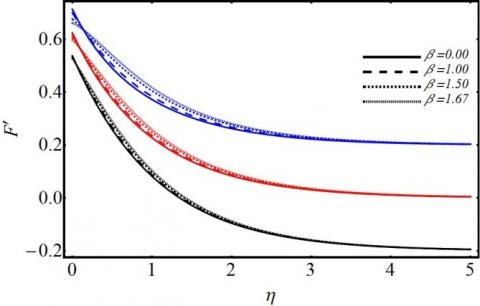Figure 2. Impact of $\beta$ on flow velocity

In Figure 2 the red colored graph represents the flow over stationary electromagnetic sheet $(\lambda=0)$. In this case when pressure gradient increases, the flow velocity gets up surged. However, the flow velocity was low for Blasius flow $(\beta=0)$ and it enhances in response to favorable pressure gradient $(\beta>0)$. This means that pressure gradient along with inertial force reduces the viscous force offered by the moving fluid. As a consequence, accelerated fluid motion takes place in the flow domain. In the Figure 2 the blue colored figure indicates the flow over a moving electromagnetic sheet (the sheet is moving in the direction of free stream velocity). In this case, increase in pressure gradient leads to accelerated fluid motion. In the absence of pressure gradient $(\beta=0)$ the Blasius flow is slow and the related velocity boundary layer grows. This is because the inertial force is dominated by viscous force due to the relative motion of the electromagnetic sheet and fluid. However, favorable pressure gradients $(\beta=1, \beta=1.5, \beta=1.67)$ yield similar fashionable accelerated motion compared to that associated with stationary electromagnetic sheet $(\lambda=0)$. Lastly, in Figure 2, the black colored figure implicates the flow over a moving electromagnetic sheet where the sheet moves in the opposite direction of free stream velocity. In this environment, Blasius flow $(\beta=0)$ provides slow motion. As $\beta$ increases (favorable pressure gradient, for instance, $(\beta=1, \beta=1.5, \beta=1.67)$), the similar type accelerated fluid motion is attained. On comparison, the velocity variation is more prominent near the sheet (irrespective stationary or moving) and asymptotic towards the ambient. On further comparison, we observe that the velocity variation is more significant when the electromagnetic sheet moves in the direction of free stream velocity $(\lambda=0.2)$.

## 3.jpgFigure 3. Impact of Z on flow velocity

Quite understandably, Figure 3 narrates the variation of flow velocity in response to different strength of modified Hartmann number Z. Nevertheless, the condition of the electromagnetic sheet (the sheet moves in the direction of $U_{\infty}(x) : \lambda=0.2$ or the sheet moves in the opposite direction of $U_{\infty}(x) : \lambda=-0.2$ or stationary sheet: $\lambda=0$), enhanced modified Hartmann number Z leads to accelerated fluid motion in the respective velocity boundary layer. However at fixed Z (for instance Z=1), the fluid velocity gets augmented in the order, $\lambda=-0.2$ (electromagnetic sheet moves in opposite direction of $\left.U_{\infty}(x)\right), \quad \lambda=0$ (stationary electromagnetic sheet) and $\lambda=0.2$ (sheet moves in the direction of $U_{\infty}(x)$). Other features of velocity profiles retain the same as shown in Figure 1.

## 4.jpg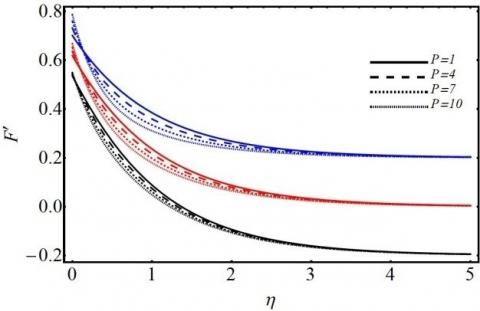Figure 4. Impact of P on flow velocity

Figure 4 portrays the velocity profiles for different porosity parameter P with respect to different conditions of the electromagnetic sheet (moving/stationary). This figure reveals that increasing porosity parameter decelerates the fluid motion. The rationale behind is that the resistive force offered by introduction of porous matrix undermines the fluid velocity and hence restrains the fluid motion. This scenario is visualized in the fixed electromagnetic sheet ($\lambda=0$), and moving electromagnetic sheet ($\lambda=-0.2 \& \lambda=0.2$). However, the visualization implicates the significant velocity variation (decelerated fluid motion) for the flow over the electromagnetic plate moving in the direction of $U_{\infty}(x)$ $(\lambda=0.2)$. Having a look into three conditions of the electromagnetic sheet, we observe that decline of velocity profiles is prominent near the sheet and approaching asymptotic behavior towards the ambient.

## 5.jpgFigure 5. Impact of $\gamma$ on flow velocity

## 6.jpg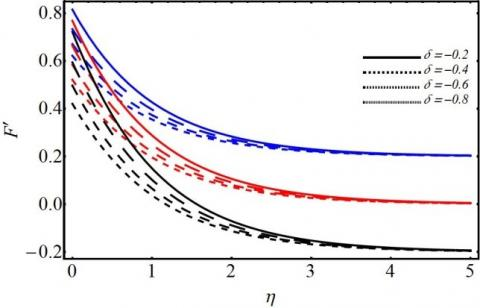Figure 6. Impact of $\delta$ on flow velocity

Figure 5 demonstrates the behavior of fluid velocity for different first order slip parameter $\gamma$. It has meaning that with augmentation of the slip parameter $\gamma$, the fluid velocity diminishes gradually. As a consequence, the wall velocity gradient reduces. Also, the velocity boundary layer becomes thick due to such effect. Figure 6 implicates that the second order slip causes to enhance the velocity distribution in any circumstance of the sheet (stationary/moving). It is pertinent to mention here that Figure 7 displays the role of suction parameter S on flow velocity. We see that it influences the boundary layer thickness adversely. The flow seems to decelerate with increasing S (Negative values of S shows injection). Suction causes the boundary layer to stick to the wall and hence, reduces the momentum. It is remarkable to note that at $\eta=0.5$, suction and injection have no significant roles on the flow velocity within the boundary layer.

Figure 8 represents the graphical variation of non-dimensional fluid temperature in response to different porosity parameter P. It is envisioned from the concerned figure that rise in porosity parameter upsurges the fluid temperature and improves the related thermal boundary layer. The fundamental cause for it is that the introduction of porous matrix slows down the fluid motion which in turn augments the temperature. Figure 9 illustrates the fluid temperature characteristics for different Prandtl fluids (fluids with Pr = 1, 2, 3, 4 for three different conditions of the electromagnetic sheet. Gazing at the figure one can declare that increase in Pr number degrades the thermal boundary layer. As a consequence, the heat transfer rate from the electromagnetic sheet would be enhanced. The rationally behind it is that the higher the Prandtl fluid, lower is the heat diffused into the boundary layer there by declines the fluid temperature.

## 7.jpg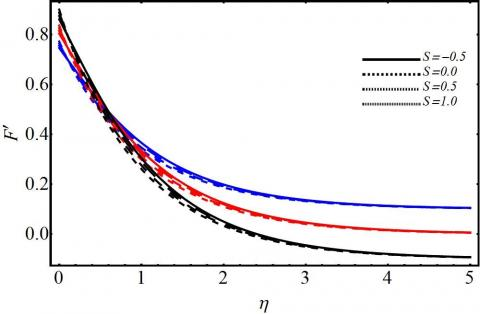Figure 7. Impact of S on flow velocity

## 8.jpg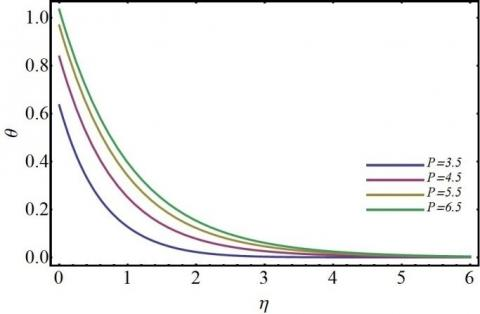Figure 8. Impact of P on temperature

Figure 10 describes the variation of skin friction in response to porosity parameter in absence as well as presence of pressure gradient. Referred to this figure it is observed that increase in pressure gradient augments the wall shear stress. However, the wall shear stress takes a lower value for Blasius flow and that avail higher values for favorable pressure gradient (for instance, $\beta=1, \beta=1.5, \beta=1.67$) at every value of porosity parameter (in the presence of porous matrix). Figure 11 depicts the variation of local skin friction for different conditions of the sheet in presence of Lorentz force due to electromagnetic field of the arrangement made on the sheet. As $\lambda$ increases, the skin friction value enhances. This figure has reflected the impact of a reduction in wall shear stress for the electromagnetic sheet moving in opposite direction of $U_{\infty}(x)(\lambda=0.2)$. The value of wall shear stress gets hiked when the electromagnetic sheet becomes stationary $(\lambda=0)$. On the other hand, when the sheet moves in the direction of $U_{\infty}(x) \quad(\lambda=0.2, \lambda=0.5)$ the wall shear stress gets enhanced at all finite strength of Lorentz force significantly.

Figure 12 focuses the Nusselt number variation for different Prandtl fluids in presence of porous matrix. As Pr enhances, thermal diffusivity gets lowered indicating low propagation of heat into the fluid leading to the decline of fluid temperature. As a result, heat transfer rate gets augmented yielding more cooling of the electromagnetic sheet (even in the presence of porous matrix). Figure 13 tells about the heat transfer rate scenario for various Prandtl fluids at different stages of pressure gradient. It is obvious from the figure that higher Prandtl fluids (Pr=3, 5, 7) cause more heat transfer rate and hence more cooling from the electromagnetic sheet subject to favorable pressure gradient $(\beta>0)$.

In all the graphs Blue lines are drawn for λ=0.2, Red lines are for λ=0.0 and Black lines for λ= -0.2.

## 9.jpgFigure 9. Impact of Pr on temperature

## 10.jpgFigure 10. Impact of β on skin friction vs P

## 11.jpg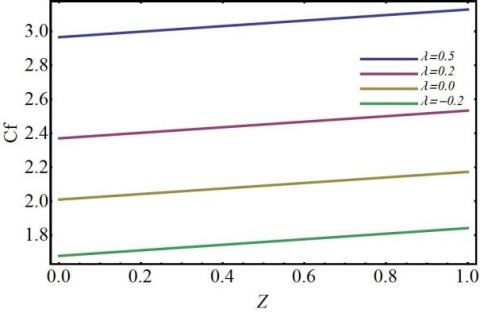Figure 11. Impact of $\lambda$ on skin friction vs Z

## 12.jpgFigure 12. Impact of Pr on Nusselt number vs P

## 13.jpg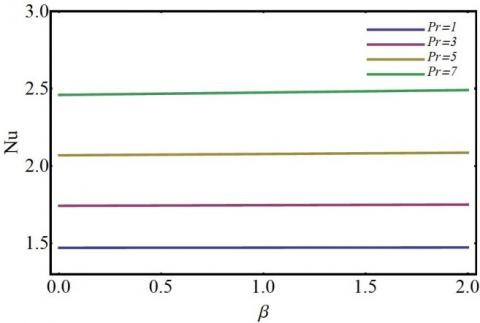Figure 13. Impact of Pr on Nusselt number vs $\beta$

5. Conclusion

The present article conveys us the investigation about the influence of second order slip and variable suction on non-linear stagnation point flow of Alumina-Water nanofluid over electromagnetic sheet embedded in a porous medium. The observed significant results of the study are briefly summarized as follows:

• Nevertheless, the electromagnetic sheet is stationary or moving in the same or the opposite direction to the free stream velocity, an accelerated fluid motion is accomplished in response to favorable pressure gradient.
• Irrespective of the situation of the electromagnetic sheet (stationary/moving), modified Hartmann number belittles the velocity boundary layer thickness.
• Introduction of porous matrix widens the velocity boundary layer subject to stationary/moving electromagnetic sheet.
• Increased first order slip reduces the fluid velocity in the entire flow domain.
• Augmented porosity parameter improves the thermal boundary layer.
• Enhanced pressure gradient upsurges the wall shear stress for stationary electromagnetic sheet, however, upsurges more significantly for the moving electromagnetic sheet.
Recommendations for future Studies

The present study can be extended with the implementation of Darcy-Forchheimer flow model, Cattaneo-Christov thermal and solutal diffusion model and entropy generation.

References

 Choi, S.U.S. (1995). Enhancing thermal conductivity of fluids with nanoparticles. ASME Fluids Eng. Division, 231: 99-105.

 Xuan, Y.M., Li, Q. (2003). Investigation on convective heat transfer and flow features of nanofluids. J. Heat Transfer, 125(1): 151-155. https://doi.org/10.1115/1.1532008

 Khan, W., Pop, I. (2010). Boundary-layer flow of a nanofluid past a stretching sheet. International Journal of Heat and Mass Transfer, 53(11-12): 2477-2483.  https://doi.org/10.1016/j.ijheatmasstransfer.2010.01.032

 Nayak, M.K., Shaw, S., Chamkha, A.J. (2018). Impact of variable magnetic field and convective boundary condition on a stretched 3D radiative flow of Cu-H2O nanofluid. Modeling Measurement and Control B, 86(3): 658-678. https://doi.org/10.18280/mmc_b.860305

 Zeeshan, A., Baig, M., Ellahi, R., Hayat, T. (2014). Flow of viscous nanofluid between the concentric cylinders. Journal of Computational and Theoretical Nanoscience, 11(3): 646-654. https://doi.org/10.1166/jctn.2014.3408

 Akbar, N.S., Nadeem, S., Haq, R.U., Khan, Z.H. (2013). Numerical solutions of Magneto-hydrodynamic boundary layer flow of tangent hyperbolic fluid towards a stretching sheet. Indian Journal of Physics, 87(11): 1121-1124. https://doi.org/10.1007/s12648-013-0339-8

 Nayak, M.K., Akbar, N.S., Pandey, V.S., Khan, Z.H., Tripathi, D. (2017). 3D free convective MHD flow of nanofluid over permeable linear stretching sheet with thermal radiation. Powder Technol., 315: 205-215. https://doi.org/10.1016/j.powtec.2017.04.017

 Nayak, M.K. (2017). MHD 3D flow and heat transfer analysis of nanofluid by shrinking surface inspired by thermal radiation and viscous dissipation. Int. J. Mech. Sci., 124: 185-193. https://doi.org/10.1016/j.ijmecsci.2017.03.014

 Roy, M., Biswal, P., Roy, S., Basak, T. (2017). Heat flow visualization during mixed convection within entrapped porous triangular cavities with moving horizontal walls via heatline analysis. Int. J Heat Mass Transf., 108: 468-489. https://doi.org/10.1016/j.ijheatmasstransfer.2016.10.114

 Khan, W.A., Makinde, O.D., Khan, Z.H. (2016). Non-aligned MHD stagnation point flow of variable viscosity nanofluids past a stretching sheet with radiative heat. International Journal of Heat and Mass Transfer, 96: 525-534. https://doi.org/10.1016/j.ijheatmasstransfer.2016.01.052

 Pantokratoras, A., Magyari, E. (2009). MHD free-convection boundary-layer flow from a Riga-plate. J Eng. Math., 64(3): 303-315. https://doi.org/10.1007/s10665-008-9259-6

 Ahmad, A., Asghar, S., Afzal, S. (2016). Flow of nanofluid past a Riga-plate. J. Magn. Magn. Mater., 402: 44-48. https://doi.org/10.1016/j.jmmm.2015.11.043

 Ahmad, R., Mustafa, M., Turkyilmazoglu, M. (2017). Buoyancy effects on nanofluid flow past a convectively heated vertical Riga-plate: A numerical study. Int. J. Heat Mass Transf., 111: 827-835. https://doi.org/10.1016/j.ijheatmasstransfer.2017.04.046

 Nayak, M.K., Shaw, S., Makinde, O.D., Chamkha, A.J. (2018). Effects of Homogenous–Heterogeneous reactions on radiative NaCl-CNP nanofluid flow past a convectively heated vertical Riga plate. J. Nanofluids, 7(4). https://doi.org/10.1166/jon.2018.1501

 Torabia, M., Torabi, M., Ghiaasiaanb, S.M., Peterson, G.P. (2017). The effect of Al2O3-water nanofluid on the heat transfer and entropy generation of laminar forced convection through isotropic porous media. Int. J. Heat Mass Transf., 111: 804–816. https://doi.org/10.1016/j.ijheatmasstransfer.2017.04.041

 Govender, S. (2017). Thermal instability in a nanofluid saturated horizontal porous layer subjected to g-gitter. Int. J. Heat Mass Transf., 110: 63-67. https://doi.org/10.1016/j.ijheatmasstransfer.2017.03.018

 Ahmed, S., Zueco, J., González, L.M.L. (2017). Numerical and analytical solutions for magneto-hydrodynamic 3D flow through two parallel porous plates. Int. J. Heat Mass Transf., 108: 322-331. https://doi.org/10.1016/j.ijheatmasstransfer.2016.11.102

 Akbar, N.S., Khan, Z.H., Nadeem, S. (2014). The combined effects of slip and convective boundary conditions on stagnation point flow of CNT suspended nanofluid over a stretching sheet. J. of Mol. Liq., 196: 21-25. https://doi.org/10.1016/j.molliq.2014.03.006

 Turkyilmazoglu, M. (2011). Multiple solutions of heat and mass transfer of MHD slip flow for the viscoelastic fluid over a stretching sheet. Int. J. Therm. Sc., 50(11): 2264-2276. https://doi.org/10.1016/j.ijthermalsci.2011.05.014

 Pandey, A.K., Kumar, M. (2017). Natural convection and thermal radiation influence on nanofluid flow over a stretching cylinder in a porous medium with viscous dissipation. Alexandria Eng. J., 56(1): 55-62. https://doi.org/10.1016/j.aej.2016.08.035

 Seddeek, M.A., Odda, S.N., Akl, M.Y., Abdelmeguid, M.S. (2009). Analytical solution for the effect of radiationon flow of a magneto-micropolar fluid past a continuously moving plate with suction and blowing. Computational Materials Science, 45(2): 423-428. https://doi.org/10.1016/j.commatsci.2008.11.001

 Nayak, M.K. (2016). Chemical reaction effect on MHD viscoelastic fluid over a stretching sheet through porous medium. Meccanica, 51(8): 1699-1711. https://doi.org/10.1007/s11012-015-0329-3

 Rashidi, M.M., Abdul Hakeem, A.K., Vishnu Ganesh, N., Ganga, B., Sheikholeslami, M., Momoniat, E. (2016). Analytical and numerical studies on heat transfer of a nanofluid over a stretching/shrinking sheet with second-order slip flow model. Int. J. of Mech. and Mat. Eng., 11: 1. https://doi.org/10.1186/s40712-016-0054-2

 Ho, C.J., Li, W.K., Chang, Y.S., Lin, C.C. (2010). Natural convection heat transfer of alumina-water nanofluid in vertical square enclosures: An experimental study. Int. J. Therm. Sci., 49(8): 1345-1353. https://doi.org/10.1016/j.ijthermalsci.2010.02.013

 Sheremet, M.A., Pop, I., Mahian, O. (2018). Natural convection in an inclined cavity with time-periodic temperature boundary conditions using nanofluids: Application in solar collectors. Int. J. Heat Mass Transf. 116: 751-761. https://doi.org/10.1016/j.ijheatmasstransfer.2017.09.070

 Mastorakis, N.E. (2005). Numerical solution of non-linear ordinary differential equations via Collocation Method (Finite Elements) and Genetic Algorithm. Proceedings of the 6th WSEAS Int. Conf. on EVOLUTIONARY COMPUTING, Lisbon, Portugal, June 16-18, 2005, pp. 36-42.